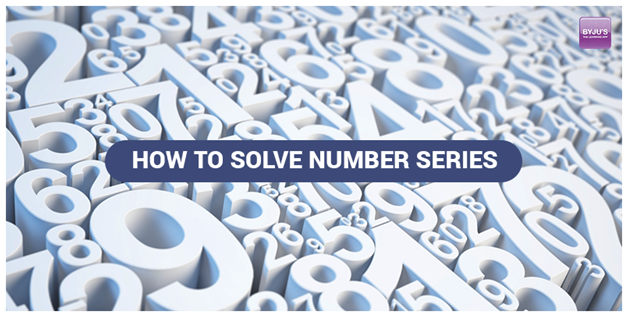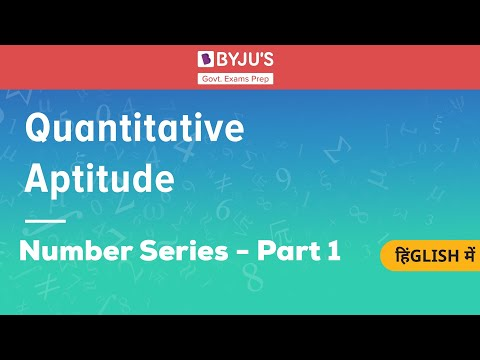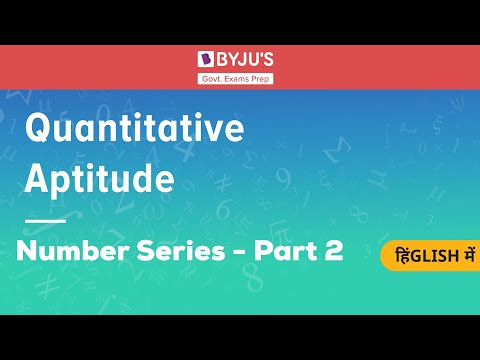# Steps To Solve Number Series In Bank Exams

Number series is one of the most common topics from the Quantitative aptitude section from which questions are asked in the various Bank exams.

There are three mains bank exams which are conducted in the country. These include:

Reasoning Ability is one of the most important sections of any bank exam. In addition, a very scoring topic of the banking syllabus under the reasoning section is Number Series.

For upcoming bank exams like SBI PO, SBI Clerk, IBPS PO, IBPS Clerk and other Govt exams, aspirants should be proficient in the Reasoning Ability section to excel in the exam.

There are two posts for which the bank exams are mostly conducted. This includes:Candidates preparing for the various bank exams can refer to the below-mentioned links to ace the quantitative aptitude section:

## What is Number Series

The arrangement of numbers in a certain order, and in which some numbers are placed wrongly in the series and some numbers are missing is called a number series.

Based on calculating the addition and subtraction of the numbers, candidates have to observe and find the accurate number to the series of numbers.

 Practise number series questions and solve them within lesser time with the free online mock test series and previous year bank exam question papers. Get more Number Series questions for bank exams in the links given below:

### Steps To Solve Number Series

Here we are providing some steps on how to solve number series question that forms an important part of the reasoning section of the banking syllabus:

Step 1: Screening

Candidates should first read the question carefully. Sometimes reading the question carefully can be enough for the candidate to solve the question.

Example:

1, 3, 7, 13, 21, 21, 31, ?

In this series, the terms are increasing by +2, +4, +6, +8, +10. So, the next term will be increased by 12. Hence, the next term is 31 + 12 = 43.

2, 4, 8, 16, 32, 64, ?

In this series, the terms are increasing by the multiples of 2. Therefore, the next term will be 64 * 2 = 128.

To learn 3 Sutras To Prepare For Reasoning Ability, check at the linked article.

Get the detailed section-wise bank exam syllabus at the linked article.

Step 2: Check the pattern Decreasing or Increasing or Alternating.

Sometimes candidates fail to decipher the pattern of the number series. During bank exam preparation, candidates should try to find out the trend of the series by looking at the series pattern. They can find it by checking the series whether it is decreasing or increasing or it is following an alternate pattern.

Example:

• 5, 10, 13, 26, 29, 58

Clearly, we can see that it is an increasing pattern.

• 729, 512, 343, 216, 125

Here, we can see that the series is of decreasing pattern.

• 4, -8, 16, -32, 64, -128

This series is neither increasing nor decreasing. So clearly, it is following an alternating pattern.

Candidates can explore more articles related to banking syllabus such as SBI PO Syllabus.

Step 3: Find the rate of increase or decrease if the series is of increasing or decreasing pattern.

During bank exam preparation, candidates should read the question carefully and find whether the nature of the series is increasing or decreasing. Moreover, on the basis of that, they should find the rate at which the terms are increasing or decreasing.

Get the IBPS Clerk prelims syllabus at the linked article and prepare yourself accordingly for the upcoming clerical cadre exam.

For a better understanding as to how to solve a number series either wrong series or a missing number series, candidates can refer to the video given below:### Types of Questions on Number Series

Here are some of the series patterns which are discussed below:

• Based on subtraction and addition

In the series 4, 9, 14, 19, 24, 29 we can see that it is an increasing series and the rate of increase is 9-4 = 5.

In the series 13, 11, 9, 7, 5 we can see that it is a decreasing series and the rate of decrease is 13-11 = 2

• Based on division and multiplication

In the series 4, 8, 16, 32, 64, we can see that it is an increasing series and the series is increasing by the multiple of 2.

In the series 100, 50, 25, 5, 1 we can see that it is a decreasing series and the series is decreasing as the terms are divided by 5.

• Based on square and cube

In the series 8, 27, 125, 343, 1331, 2197, we can see that it is an increasing series. The series is the cube of the consecutive prime numbers from 2 to 13.

The table given below mentions a few other Quantitative Aptitude topics which candidates need to excel in to crack the upcoming bank exams. Aspirants can visit the articles below and strengthen their command over the numerical ability section:

Step 4: Check if the series is alternating

For alternating series, candidates need to check whether the series has two different operations, which are being alternately performed, or there is a mix of two series.

Example:

In the series 4, 9 6, 18, 9, 27, 13, 36. This series is a mix of two series 9, 18, 27, 36 and 4, 6, 9, 13.

In the series 200, 600, 1200, 1600, 3200, 3600, 7200 two types of operations are going, one is the addition of 400 and the second is multiplication by 2.

### Sample Questions – Number Series

Candidates must know that number series is one of the easiest topics in the numerical ability section for bank exams and more practice will help them score more and solve them within a shorter period of time.

Given below a few sample questions for the reference of candidates and to help them prepare for the upcoming banking sector examinations.

Directions (Q1 – Q3): For the given number series, find what will come in place of the question mark (?)

Q 1. 2 10 50 ? 1250 6250

1. 125
2. 450
3. 100
4. 250
5. 150

Solution:

2×5 = 10

10×5 = 50

50×5 = 250

250×5 = 1250

1250×5 = 6250

Q 2. 5 5 7.5 ? 37.5 112.5

1. 10.5
2. 12
3. 15
4. 15.5
5. 17

Solution:

5×1 = 5

5×1.5 = 7.5

7.5×2 = 15

15×2.5 = 37.5

37.5×3 = 112.5Q 3. 15 19 28 ? 69 105

1. 44
2. 46
3. 45
4. 47
5. 48

Solution:

15 + 22 = 19

19 + 32 = 28

28 + 42 = 44

44 + 52 = 69

69 + 62 = 105

Directions (Q4 – Q6): In the following series, find which of the given number is wrong and does not follow the pattern:

Q 4. 564 282 140 70.5 35.25

1. 564
2. 140
3. 35.25
4. 70.5
5. 281

Solution:

564/2 = 282

282/2 = 141

141/2 = 70.5

70.5/2 = 35.25

Q 5. 633 624 598 534 409 193

1. 193
2. 409
3. 624
4. 534
5. 598

Solution:

633 – 23 = 625

625 – 33 = 598

598 – 43 = 534

534 – 53 = 409

409 – 63 = 193

Q 6. 17 85 425 2115 10625 53125

1. 17
2. 85
3. 425
4. 2115
5. 53125

Solution:

17×5 = 85

85×5 = 425

425×5 = 2125

2125×5 =  10625

10625×5 = 53125

Questions in the form of wrong number series and missing number series are only asked in the bank exams. Most importantly a candidate must improve their calculation speeds to be able to answer the number series questions.

To know more about SBI Clerk Syllabus, check at the linked article.

Apart from the Quantitative Aptitude section, candidates must also focus equally well on the other sections which are a part of the bank exam syllabus. Candidates can refer to the table given below for the detailed section-wise syllabus for bank exams:

Therefore, we hope that with lots of practice, these steps can help the candidates during their bank exam preparation to build the intuition that is required to solve number series problems during the bank exams.

Stay tuned with BYJU’S for more updated content on bank exams preparation.

To watch the continued part of the video based on the conceptual knowledge of Number series and the type of questions asked from this topic, candidates can check the video given below: